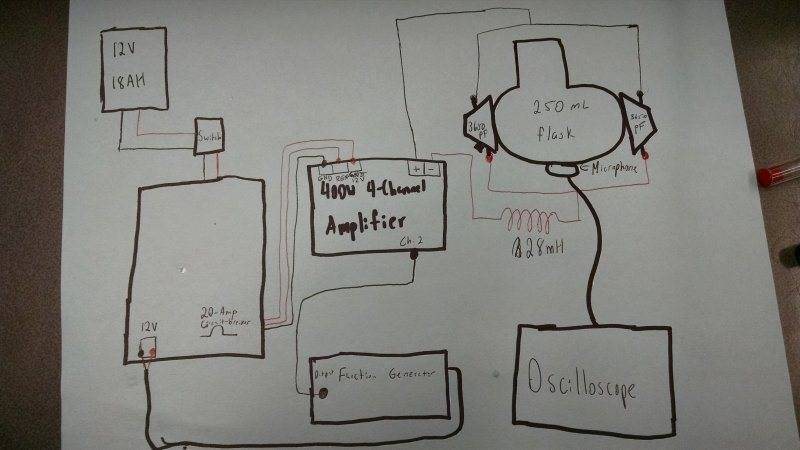# Why does my inductor coil have no effect on my circuit

I have the circuit with a drawing below, to create sonoluminescence. One of the steps needed was an inductor coil in order to achieve electrical resonance within the circuit. The link provided here: http://www.macgeisler.de/nld/sbsl-howto.html told me to find the inductance needed through the equation L=1/C(6.28(f)2) Where L is inductance, C is the capacitance of my transducers, he said to look at them as a parallel plate capacitor, but I just found the static capacitance on the website I bought it from which on the website said the static capacitance was 3650pF, and the resonant frequency which was 42kHz. When I made the inductor coil for this, using an iron nail covered in electrical tape and coated copper wire, I am getting all the same results on my voltage and frequency from the oscilloscope hooked up. This is AC electricity by the way. So why is my inductor coil not working or having any effect on my circuit? A physics professor from a college I visited said it was because my frequency generator was already pumping out the tuned electricity, but shouldn't the inductor atleast raise the voltage or something? The inductance says 28mH on my picture but I made a new one that is around 80mH now. But even if the inductance is off shouldn't it still have an effect on the voltage? Thanks for the help!mfb
Mentor
How did you estimate the inductance of your coil? If it is way too small (and your description sounds like that), it won't have a relevant effect.

I measured it through the equation L=((the magnetic permetivity)(turns)^2(area of coil))/length of coil

mfb
Mentor
What was the result?

You can also check its voltage over time with the oscilloscope. If it is small compared to the source voltage, then the coil is too small.

I can check over time. About how much time would be sufficient? And other projects had ones of only around 20mH

mfb
Mentor
You have the formula to compute the required inductance in post 1. If you hit that, it should help.
Note that C is the combined capacitance from both capacitors.

If C is the capacitance of both though shouldn't my coil still work since it would be larger than needed, because a higher capacitance in the formula means a lower inductance. I calculated the capacitance as a parallel plate capacitor too and got a really high ~35H when other experiments used ~35mH

tech99
Gold Member
The inductor is in series with the capacitance of the transducers, and forms a series resonant circuit. With no inductor, the current in the circuit will be limited by the capacitive reactance of the transducers. With the correct inductor, the circuit will have zero reactance plus a small resistance and will allow a large current to flow. Due to the resonant action, when this happens, a magnified voltage will appear across the transducers. The coil you have made is probably inefficient due to eddy current losses in the iron.nail, and the inductive reactance must equal the combined capacitive reactance of the transducers. So you need a better inductor - maybe ferrite.

OK. That helps a lot. Thank you!